International
Tables for
Crystallography
Volume A
Space-group symmetry
Edited by Th. Hahn

International Tables for Crystallography (2006). Vol. A, ch. 2.2, p. 34

## Section 2.2.14.2. Projections of centred cells (lattices)

Th. Hahna* and A. Looijenga-Vosb

aInstitut für Kristallographie, Rheinisch-Westfälische Technische Hochschule, Aachen, Germany, and bLaboratorium voor Chemische Fysica, Rijksuniversiteit Groningen, The Netherlands
Correspondence e-mail:  hahn@xtl.rwth-aachen.de

#### 2.2.14.2. Projections of centred cells (lattices)

| top | pdf |

For centred lattices, two different cases may occur:

• (i) The projection direction is parallel to a lattice-centring vector. In this case, the projected plane cell is primitive for the centring types A, B, C, I and R. For F lattices, the multiplicity is reduced from 4 to 2 because c-centred plane cells result from projections along face diagonals of three-dimensional F cells.

#### Examples

 (1) A body-centred lattice with centring vectorgives a primitive net, if projected along [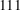], [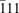], [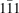] or [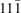]. (2) A C-centred lattice projects to a primitive net along the directions  and [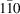]. (3) An R-centred lattice described with `hexagonal axes' (triple cell) results in a primitive net, if projected along [], [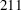] or [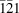] for the obverse setting. For the reverse setting, the corresponding directions are [], [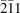], [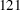]; cf. Chapter 1.2.

• (ii) The projection direction is not parallel to a lattice-centring vector (general projection direction). In this case, the plane cell has the same multiplicity as the three-dimensional cell. Usually, however, this centred plane cell is unconventional and a transformation is required to obtain the conventional plane cell. This transformation has been carried out for the projection data in this volume.

#### Examples

 (1) Projection along [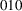] of a cubic I-centred cell leads to an unconventional quadratic c-centred plane cell. A simple cell transformation leads to the conventional quadratic p cell. (2) Projection along [] of an orthorhombic I-centred cell leads to a rectangular c-centred plane cell, which is conventional. (3) Projection along [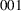] of an R-centred cell (both in obverse and reverse setting) results in a triple hexagonal plane cell h (the two-dimensional analogue of the H cell, cf. Chapter 1.2). A simple cell transformation leads to the conventional hexagonal p cell.